Browse by Topic

# New in 13: Optimization, PDEs & System Modeling

Two years ago we released Version 12.0 of the Wolfram Language. Here are the updates in optimization, PDEs and system modeling since then, including the latest features in 13.0. The contents of this post are compiled from Stephen Wolfram’s Release Announcements for 12.1, 12.2, 12.3 and 13.0.

## Mathematical Optimization

### The Leading Edge of Optimization (March 2020)

In Version 12.0 we introduced industrial-scale convex optimization. We covered most of the usual problem classes (like linear, semidefinite, quadratic and conic). But there was one straggler: geometric optimization. And now we’re adding that for 12.1: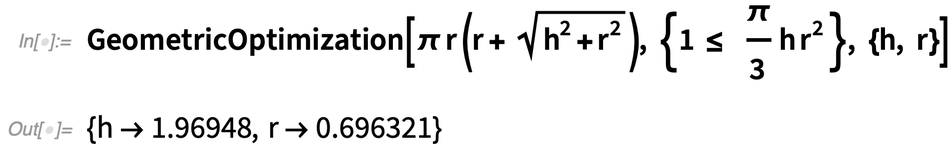✕ ```GeometricOptimization[\[Pi] r (r + Sqrt[h^2 + r^2]), {1 <= \[Pi]/ 3 h r^2 }, {h, r}]```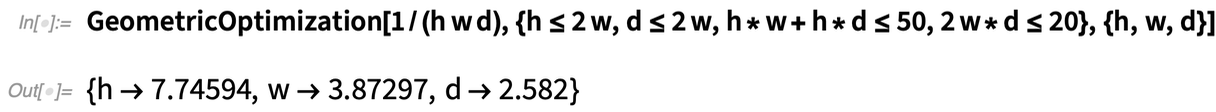✕ ```GeometricOptimization[ 1/(h w d), {h <= 2 w, d <= 2 w, h*w + h*d <= 50, 2 w*d <= 20}, {h, w, d}]```

You can solve all sorts of practical problems with GeometricOptimization—with thousands of variables if need be. As one example, consider laying out rectangles of certain sizes with a certain partial ordering in x and y. To specify the problem, you give a bunch of inequalities: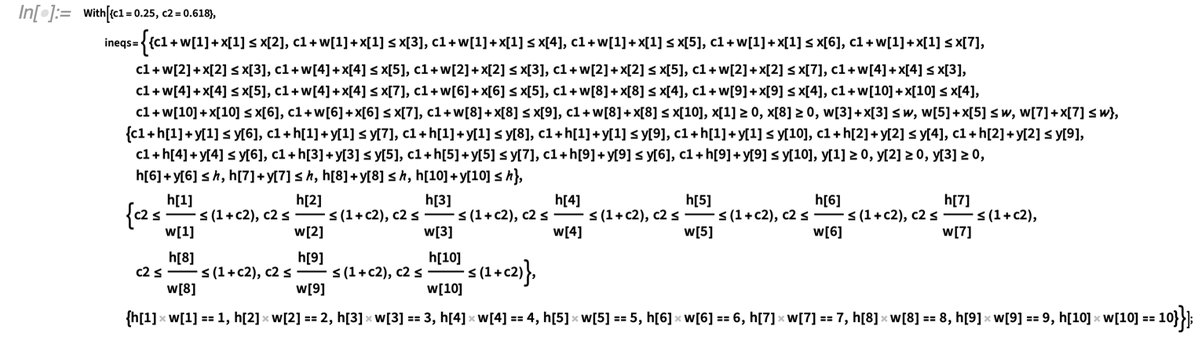✕ ```With[{c1 = 0.25, c2 = 0.618}, ineqs = {{c1 + w + x <= x, c1 + w + x <= x, c1 + w + x <= x, c1 + w + x <= x, c1 + w + x <= x, c1 + w + x <= x, c1 + w + x <= x, c1 + w + x <= x, c1 + w + x <= x, c1 + w + x <= x, c1 + w + x <= x, c1 + w + x <= x, c1 + w + x <= x, c1 + w + x <= x, c1 + w + x <= x, c1 + w + x <= x, c1 + w + x <= x, c1 + w + x <= x, c1 + w + x <= x, c1 + w + x <= x, c1 + w + x <= x, c1 + w + x <= x, x >= 0, x >= 0, w + x <= \[ScriptW], w + x <= \[ScriptW], w + x <= \[ScriptW]}, {c1 + h + y <= y, c1 + h + y <= y, c1 + h + y <= y, c1 + h + y <= y, c1 + h + y <= y, c1 + h + y <= y, c1 + h + y <= y, c1 + h + y <= y, c1 + h + y <= y, c1 + h + y <= y, c1 + h + y <= y, c1 + h + y <= y, y >= 0, y >= 0, y >= 0, h + y <= \[ScriptH], h + y <= \[ScriptH], h + y <= \[ScriptH], h + y <= \[ScriptH]}, {c2 <= h/w <= (1 + c2), c2 <= h/w <= (1 + c2), c2 <= h/w <= (1 + c2), c2 <= h/w <= (1 + c2), c2 <= h/w <= (1 + c2), c2 <= h/w <= (1 + c2), c2 <= h/w <= (1 + c2), c2 <= h/w <= (1 + c2), c2 <= h/w <= (1 + c2), c2 <= h/w <= (1 + c2)}, {h w == 1, h w == 2, h w == 3, h w == 4, h w == 5, h w == 6, h w == 7, h w == 8, h w == 9, h w == 10}}];```

It then takes only about a second to generate an optimal solution: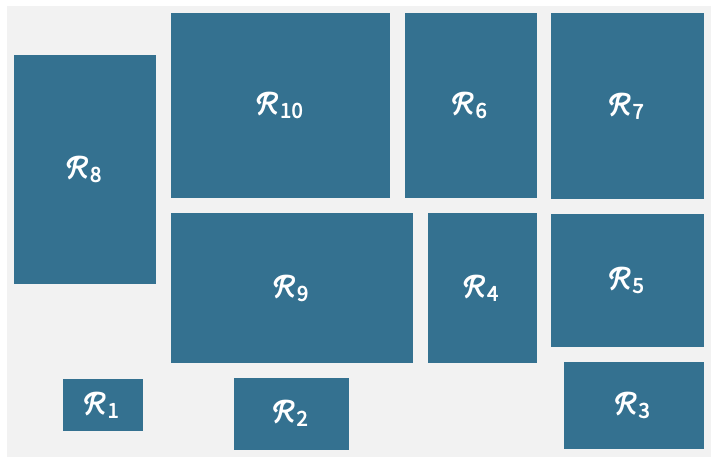In optimization, there are usually two broad types: continuous and discrete. Our convex optimization functions in 12.0 handled the case of continuous variables. But a major new feature—and innovation—in 12.1 is the addition of support for discrete (i.e. integer) variables, and for mixed discrete and continuous variables.

Here’s a very simple example: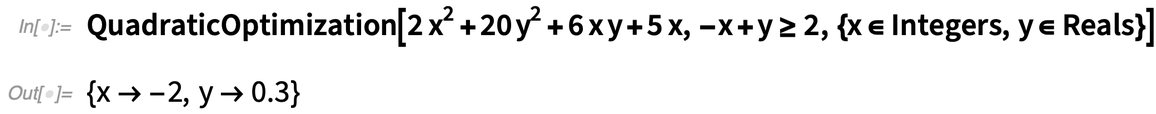✕ ```QuadraticOptimization[ 2 x^2 + 20 y^2 + 6 x y + 5 x, -x + y >= 2, {x \[Element] Integers, y \[Element] Reals}]```

If x wasn’t constrained to be an integer, the result would be different: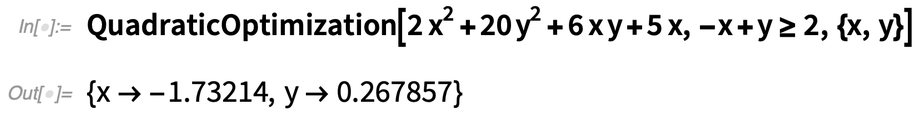✕ ```QuadraticOptimization[ 2 x^2 + 20 y^2 + 6 x y + 5 x, -x + y >= 2, {x, y}]```

But—as with our other optimization capabilities—this can be scaled up, though the combinatorial optimization that’s involved is fundamentally more computationally difficult (and for example it’s often NP-complete). And actually the only reason we can do large-scale problems of this kind at all is that we’ve implemented a novel iteration-based technique that successfully unlocks mixed convex optimization.

### The Latest in Industrial-Strength Convex Optimization (December 2020)

Starting in Version 12.0, we’ve been adding state-of-the-art capabilities for solving large-scale optimization problems. In Version 12.2 we’ve continued to round out these capabilities.

One new thing is the superfunction ConvexOptimization, which automatically handles the full spectrum of linear, linear-fractional, quadratic, semidefinite and conic optimization—giving both optimal solutions and their dual properties. In 12.1 we added support for integer variables (i.e. combinatorial optimization); in 12.2 we’re also adding support for complex variables.

But the biggest new things for optimization in 12.2 are the introduction of robust optimization and of parametric optimization. Robust optimization lets you find an optimum that’s valid across a whole range of values of some of the variables. Parametric optimization lets you get a parametric function that gives the optimum for any possible value of particular parameters. So for example this finds the optimum for x, y for any (positive) value of α: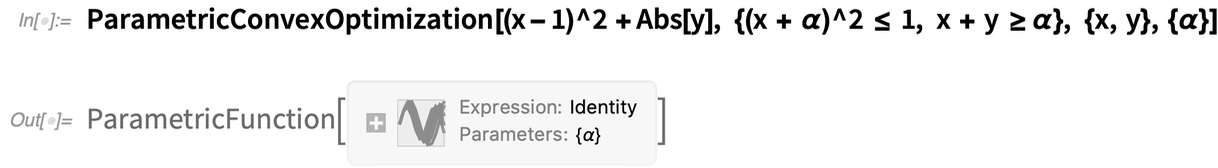✕ ```ParametricConvexOptimization[(x - 1)^2 + Abs[y], {(x + \[Alpha])^2 <= 1, x + y >= \[Alpha]}, {x, y}, {\[Alpha]}]```

Now evaluate the parametric function for a particular α: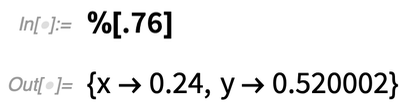✕ `%[.76]`

As with everything in the Wolfram Language, we’ve put a lot of effort into making sure that convex optimization integrates seamlessly into the rest of the system—so you can set up models symbolically, and flow their results into other functions. We’ve also included some very powerful convex optimization solvers. But particularly if you’re doing mixed (i.e. real+integer) optimization, or you’re dealing with really huge (e.g. 10 million variables) problems, we’re also giving access to other, external solvers. So, for example, you can set up your problem using Wolfram Language as your “algebraic modeling language”, then (assuming you have the appropriate external licenses) just by setting Method to, say, “Gurobi” or “Mosek” you can immediately run your problem with an external solver. (And, by the way, we now have an open framework for adding more solvers.)

### Symbolic Optimization Breakthrough (May 2021)

A major step forward in Version 12.0 was the introduction of industrial-strength convex optimization, routinely handling problems involving millions of variables in the linear case and thousands in the nonlinear case. In Version 12.0 everything had to be numerical (in 12.1 we added integer optimization). In Version 12.3 we’re now adding the possibility for symbolic parameters in large-scale linear and quadratic problems, as in this small example: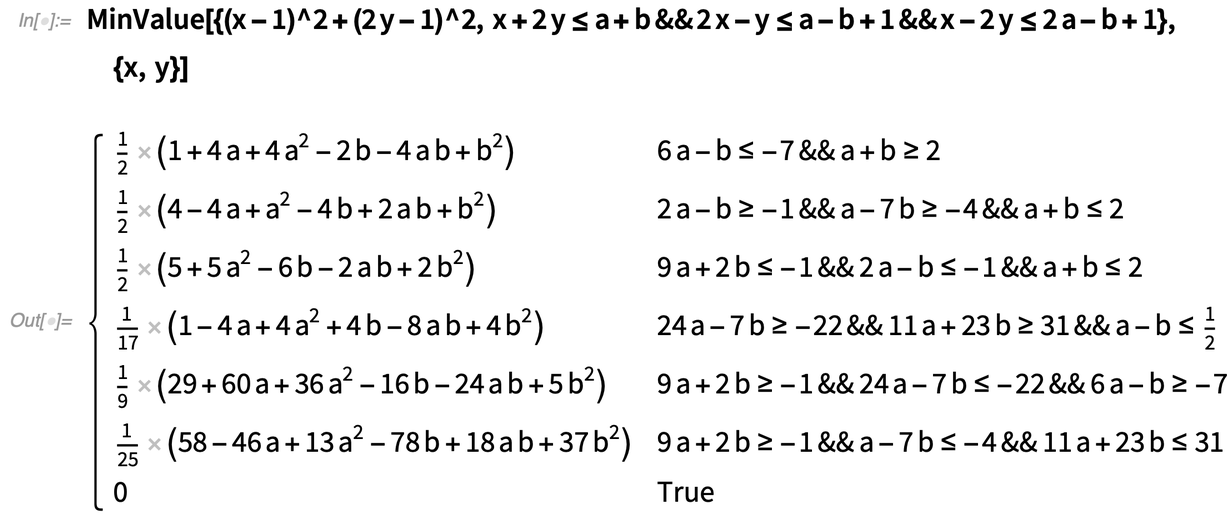✕ ```MinValue[{(x - 1)^2 + (2 y - 1)^2, x + 2 y <= a + b && 2 x - y <= a - b + 1 && x - 2 y <= 2 a - b + 1}, {x, y}]```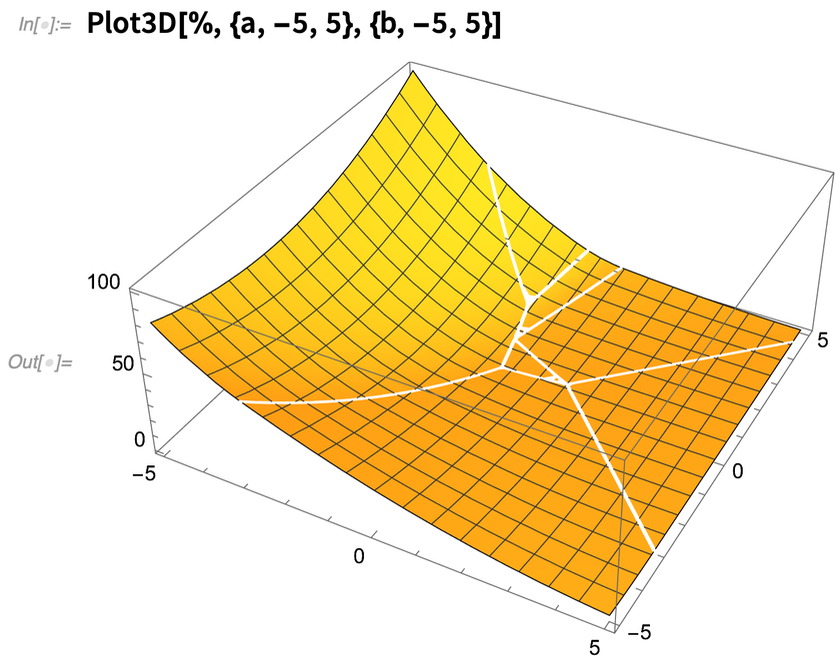✕ `Plot3D[%, {a, -5, 5}, {b, -5, 5}]`

In typical convex optimization computations not involving symbolic parameters one aims only for approximate numerical results, and it wasn’t clear whether there was any general method for getting exact numerical results. But for Version 12.3 we’ve found one, and we’re now able to give exact numerical results which you can, for example, evaluate to any precision you want.

Here’s a geometric optimization problem—which can now be solved exactly in terms of transcendental root objects: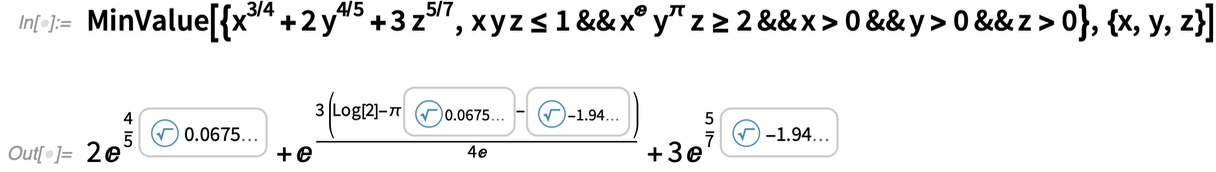✕ ```MinValue[{x^(3/4) + 2 y^(4/5) + 3 z^(5/7), x y z <= 1 && x^E y^\[Pi] z >= 2 && x > 0 && y > 0 && z > 0}, {x, y, z}]```

Given such an exact solution, it’s now possible to do numerical evaluation to any precision: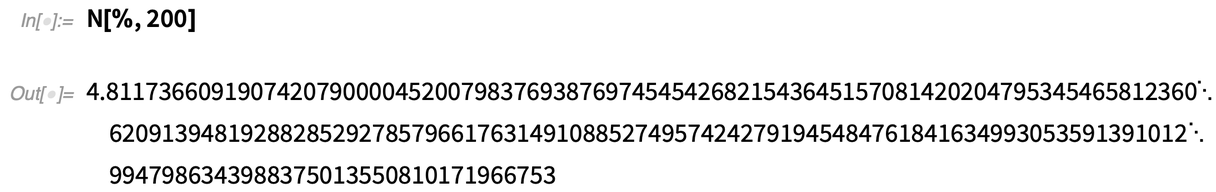✕ `N[%, 200]`

## PDE Modeling

### Convenient Real-World PDEs (December 2020)

In some ways we’ve been working towards it for 30 years. We first introduced NDSolve back in Version 2.0, and we’ve been steadily enhancing it ever since. But our long-term goal has always been convenient handling of real-world PDEs of the kind that appear throughout high-end engineering. And in Version 12.2 we’ve finally got all the pieces of underlying algorithmic technology to be able to create a truly streamlined PDE-solving experience.

OK, so how do you specify a PDE? In the past, it was always done explicitly in terms of particular derivatives, boundary conditions, etc. But most PDEs used for example in engineering consist of higher-level components that “package together” derivatives, boundary conditions, etc. to represent features of physics, materials, etc.

The lowest level of our new PDE framework consists of symbolic “terms”, corresponding to common mathematical constructs that appear in real-world PDEs. For example, here’s a 2D “Laplacian term”: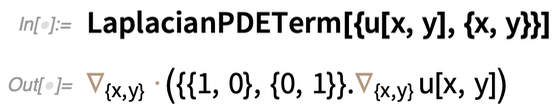✕ `LaplacianPDETerm[{u[x, y], {x, y}}]`

And now this is all it takes to find the first 5 eigenvalues of the Laplacian in a regular polygon: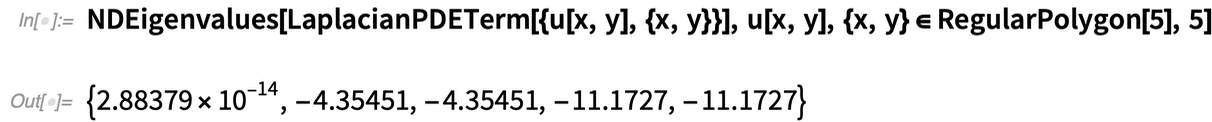✕ ```NDEigenvalues[LaplacianPDETerm[{u[x, y], {x, y}}], u[x, y], {x, y} \[Element] RegularPolygon, 5]```

And the important thing is that you can put this kind of operation into a whole pipeline. Like here we’re getting the region from an image, solving for the 10th eigenmode, and then 3D plotting the result: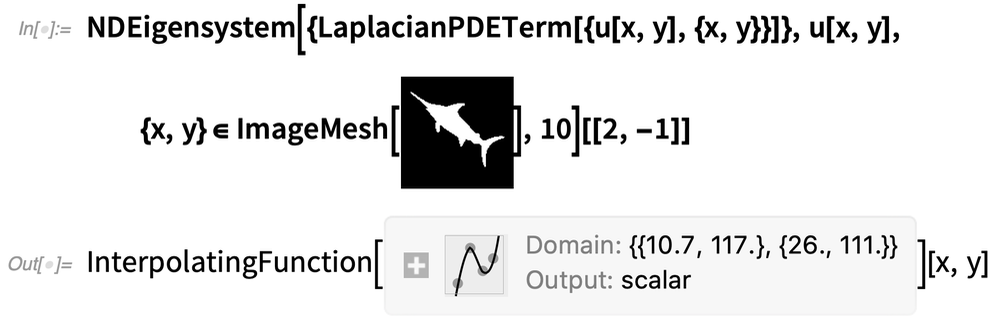✕ ```NDEigensystem[{LaplacianPDETerm[{u[x, y], {x, y}}]}, u[x, y], {x, y} \[Element] ImageMesh[CloudGet["https://wolfr.am/ROWwBtE7"]], 10][[2, -1]]```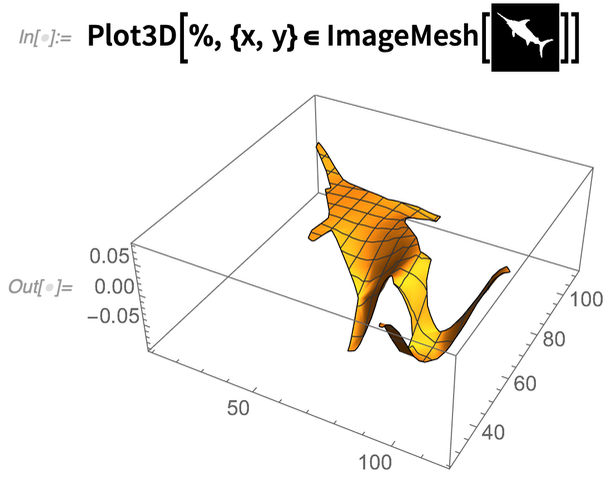✕ ```Plot3D[%, {x, y} \[Element] ImageMesh[CloudGet["https://wolfr.am/ROWwGqjg"]]]```

In addition to LaplacianPDETerm, there are things like DiffusionPDETerm and ConvectionPDETerm that represent other terms that arise in real-world PDEs. Here’s a term for isotropic diffusion with unit diffusion coefficient: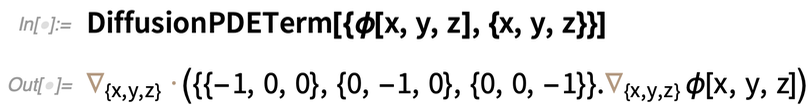✕ `DiffusionPDETerm[{\[Phi][x, y, z], {x, y, z}}]`

Beyond individual terms, there are also “components” that combine multiple terms, usually with various parameters. Here’s a Helmholtz PDE component: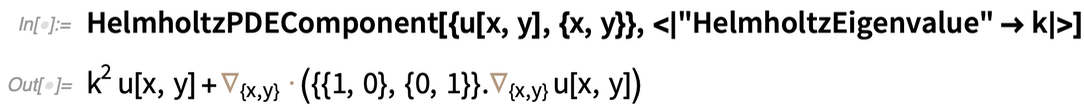✕ `HelmholtzPDEComponent[{u[x, y], {x, y}}, <|"HelmholtzEigenvalue" -> k|>]`

By the way, it’s worth pointing out that our “terms” and “components” are set up to represent the symbolic structure of PDEs in a form suitable for structural manipulation and for things like numerical analysis. And to ensure that they maintain their structure, they’re normally kept in an inactivated form. But you can always “activate” them if you want to do things like algebraic operations: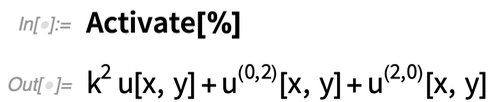✕ `Activate[%]`

In real-world PDEs, one’s often dealing with actual, physical processes taking place in actual physical materials. And in Version 12.2 we’ve got immediate ways to deal not only with things like diffusion, but also with acoustics, heat transfer and mass transport—and to feed in properties of actual materials. Typically the structure is that there’s a PDE “component” that represents the bulk behavior of the material, together with a variety of PDE “values” or “conditions” that represent boundary conditions.

Here’s a typical PDE component, using material properties from the Wolfram Knowledgebase: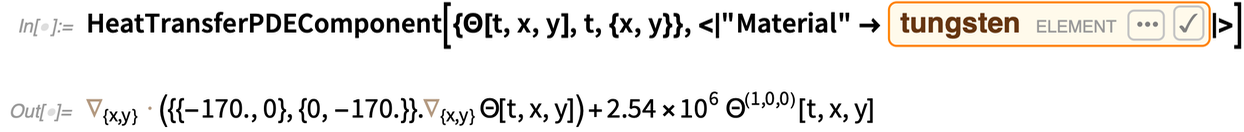✕ ```HeatTransferPDEComponent[{\[CapitalTheta][t, x, y], t, {x, y}}, <| "Material" -> CloudGet["https://wolfr.am/ROWwUQai"]|>]```

There’s quite a bit of diversity and complexity to the possible boundary conditions. For example, for heat transfer, there’s HeatFluxValue, HeatInsulationValue and five other symbolic boundary condition specification constructs. In each case, the basic idea is to say where (geometrically) the condition applies, then what it applies to, and what parameters relate to it.

So, for example, here’s a condition that specifies that there’s a fixed “surface temperature” θ0 everywhere outside the (circular) region defined by x2 + y2 = 1: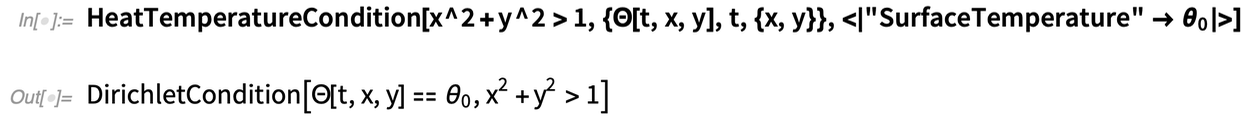✕ ```HeatTemperatureCondition[ x^2 + y^2 > 1, {\[CapitalTheta][t, x, y], t, {x, y}}, <| "SurfaceTemperature" -> Subscript[\[Theta], 0]|>]```

What’s basically happening here is that our high-level “physics” description is being “compiled” into explicit “mathematical” PDE structures—like Dirichlet boundary conditions.

OK, so how does all this fit together in a real-life situation? Let me show an example. But first, let me tell a story. Back in 2009 I was having tea with our lead PDE developer. I picked up a teaspoon and asked “When will we be able to model the stresses in this?” Our lead developer explained that there was quite a bit to build to get to that point. Well, I’m excited to say that after 11 years of work, in Version 12.2 we’re there. And to prove it, our lead developer just gave me… a (computational) spoon!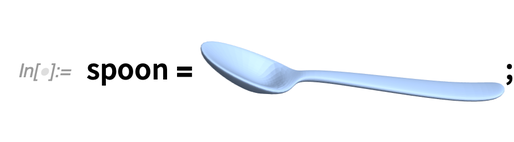✕ `spoon = CloudGet["https://wolfr.am/ROWx6wKF"];`

The core of the computation is a 3D diffusion PDE term, with a “diffusion coefficient” given by a rank-4 tensor parametrized by Young’s modulus (here Y) and Poisson ratio (ν):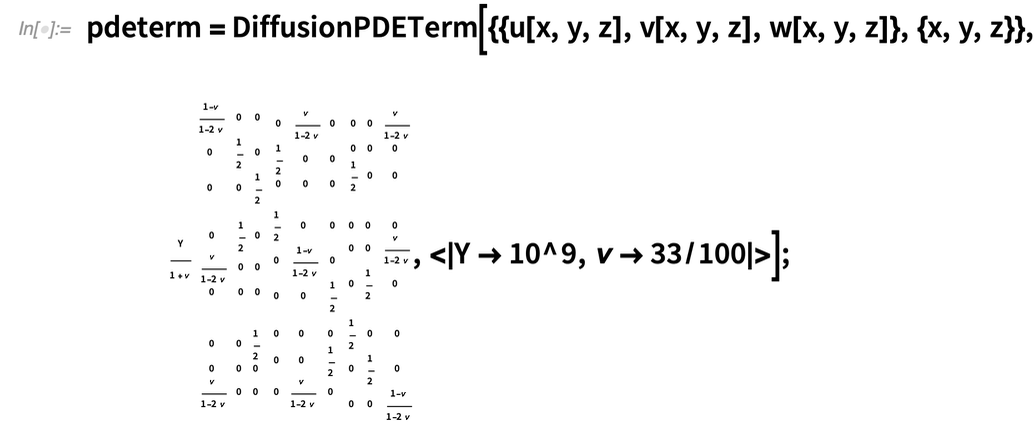✕ ```pdeterm = DiffusionPDETerm[{{u[x, y, z], v[x, y, z], w[x, y, z]}, {x, y, z}}, Y/(1 + \[Nu]) { {{ {(1 - \[Nu])/(1 - 2 \[Nu]), 0, 0}, {0, 1/2, 0}, {0, 0, 1/2} }, { {0, \[Nu]/(1 - 2 \[Nu]), 0}, {1/2, 0, 0}, {0, 0, 0} }, { {0, 0, \[Nu]/(1 - 2 \[Nu])}, {0, 0, 0}, {1/2, 0, 0} }}, {{ {0, 1/2, 0}, {\[Nu]/(1 - 2 \[Nu]), 0, 0}, {0, 0, 0} }, { {1/2, 0, 0}, {0, (1 - \[Nu])/(1 - 2 \[Nu]), 0}, {0, 0, 1/2} }, { {0, 0, 0}, {0, 0, \[Nu]/(1 - 2 \[Nu])}, {0, 1/2, 0} }}, {{ {0, 0, 1/2}, {0, 0, 0}, {\[Nu]/(1 - 2 \[Nu]), 0, 0} }, { {0, 0, 0}, {0, 0, 1/2}, {0, \[Nu]/(1 - 2 \[Nu]), 0} }, { {1/2, 0, 0}, {0, 1/2, 0}, {0, 0, (1 - \[Nu])/(1 - 2 \[Nu])} }} }, <|Y -> 10^9, \[Nu] -> 33/100|>];```

There are boundary conditions to specify how the spoon is being held, and pushed. Then solving the PDE (which takes just a few seconds) gives the displacement field for the spoon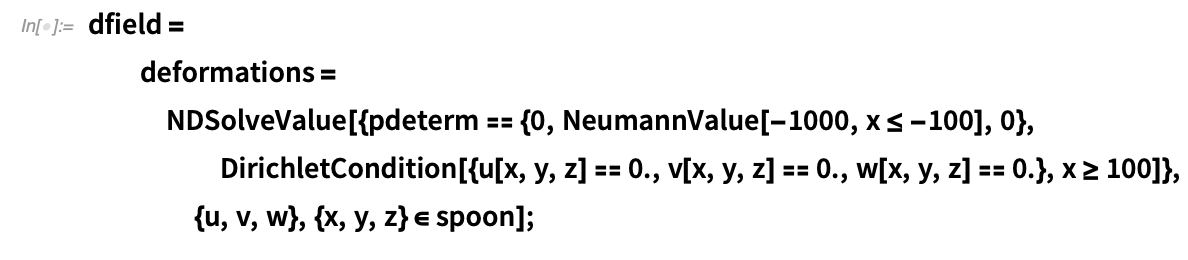✕ ```dfield = deformations = NDSolveValue[{pdeterm == {0, NeumannValue[-1000, x <= -100], 0}, DirichletCondition[{u[x, y, z] == 0., v[x, y, z] == 0., w[x, y, z] == 0.}, x >= 100]}, {u, v, w}, {x, y, z} \[Element] spoon];```

which we can then use to find how the spoon would deform: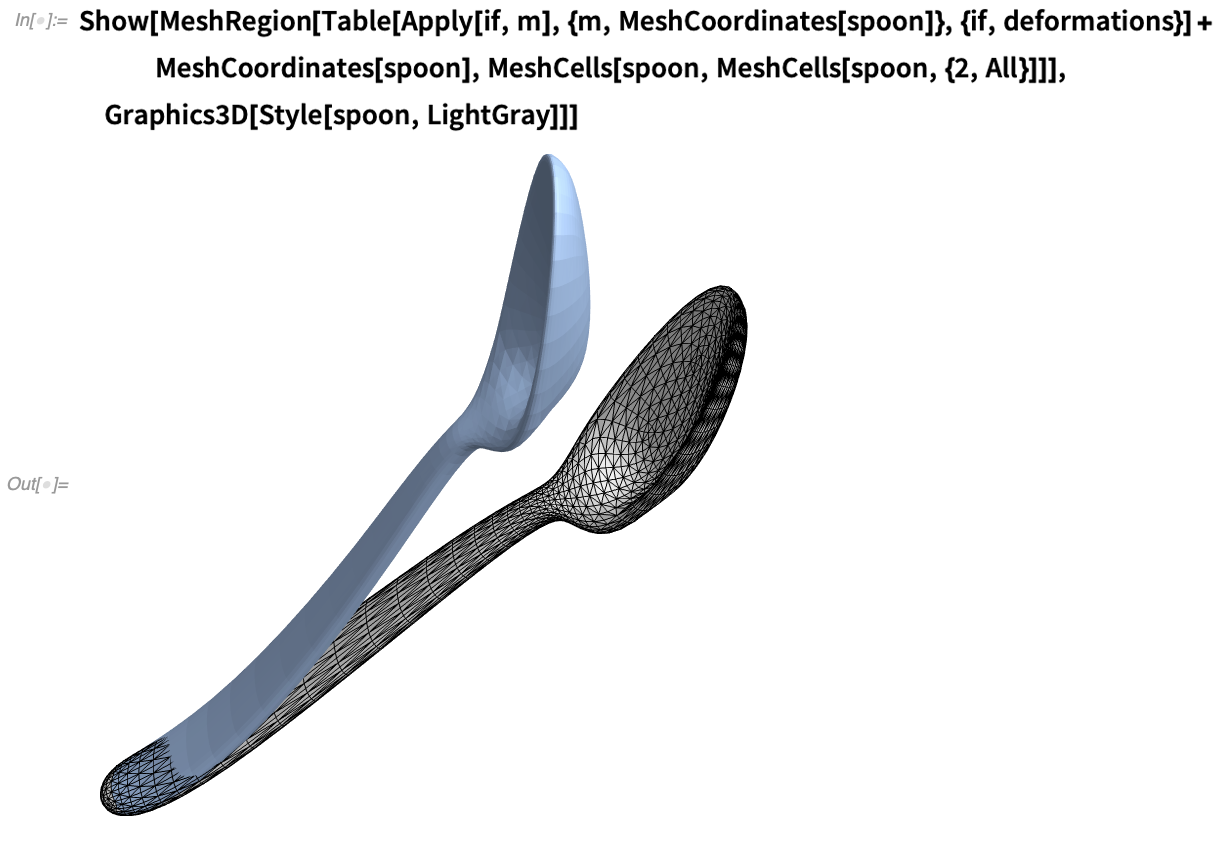✕ ```Show[MeshRegion[ Table[Apply[if, m], {m, MeshCoordinates[spoon]}, {if, deformations}] + MeshCoordinates[spoon], MeshCells[spoon, MeshCells[spoon, {2, All}]]], Graphics3D[Style[spoon, LightGray]]]```

PDE modeling is a complicated area, and I consider it to be a major achievement that we’ve now managed to “package” it as cleanly as this. But in Version 12.2, in addition to the actual technology of PDE modeling, something else that’s important is a large collection of computational essays about PDE modeling—altogether about 400 pages of detailed explanation and application examples, currently in acoustics, heat transfer and mass transport, but with many other domains to come.

### More PDE Modeling: Solid & Structural Mechanics (December 2021)

PDEs are both difficult to solve and difficult to set up for particular situations. Over the course of many years we’ve built state-of-the-art finite-element solution capabilities for PDEs. We’ve also built our groundbreaking symbolic computational geometry system that lets us flexibly describe regions for PDEs. But starting in Version 12.2 we’ve done something else too: we’ve started creating explicit symbolic modeling frameworks for particular kinds of physical systems that can be modeled with PDEs. We’ve already got heat transfer, mass transport and acoustics. Now in Version 13.0 we’re adding solid and structural mechanics.

For us a “classic test problem” has been the deflection of a teaspoon. Here’s how we can now set that up. First we need to define our variables: the displacements of the spoon in each direction at each x, y, z point: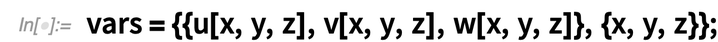✕

Then we need to say what the material parameters of our spoon are. And here we get to make use of our whole knowledgebase, which contains detailed information on many kinds of materials: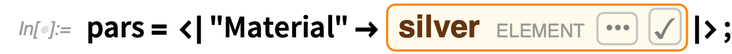✕

Now we’re ready to actually set up and solve the PDE problem: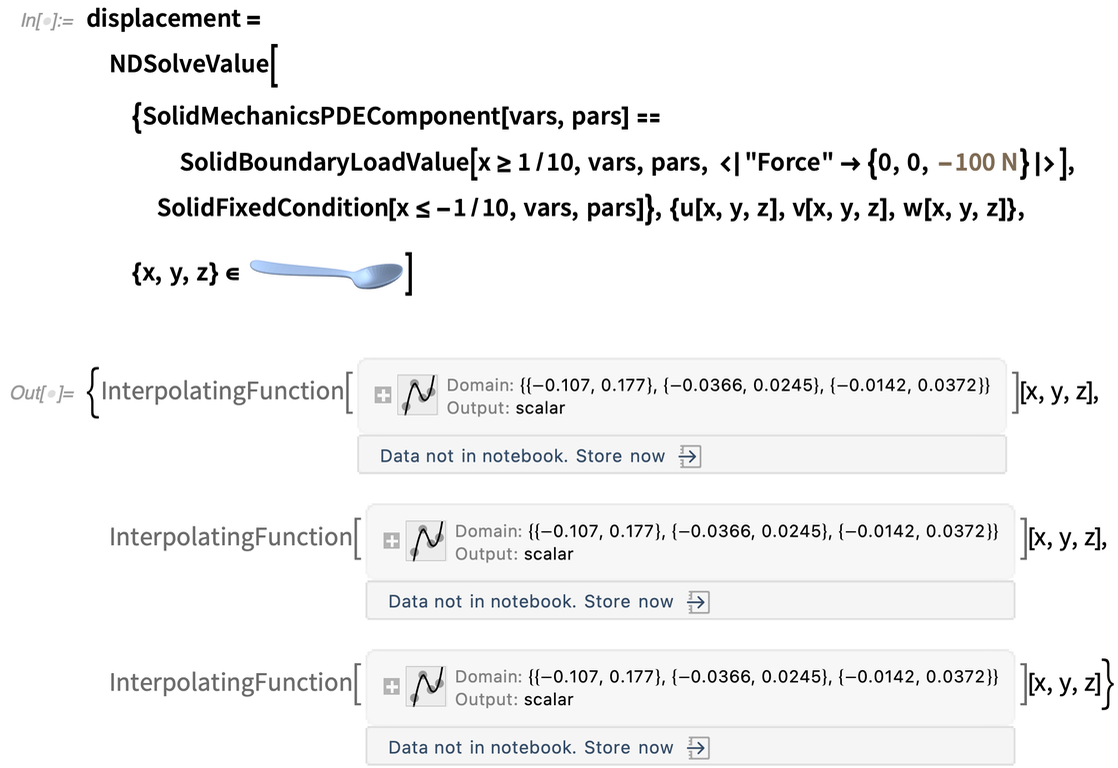✕

The result is given as a list of interpolating functions for the x, y, z displacements. Now we can use a new Version 13.0 graphics function to immediately visualize this result: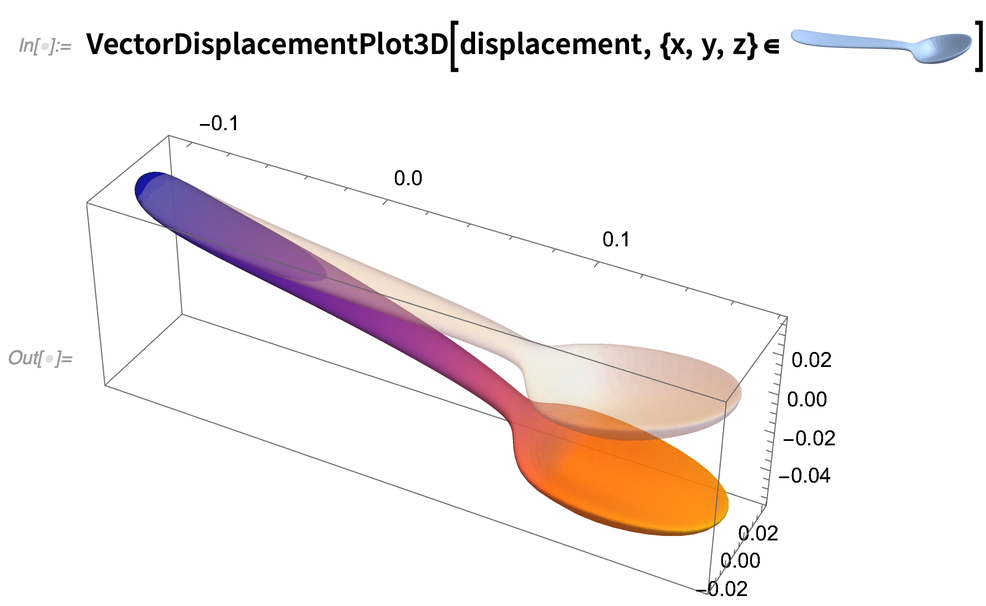✕

But conveniently packaged in those interpolation functions is also lots more detail about the solution we got. For example, here’s the strain tensor for the spoon, given as a symmetrized array of interpolating functions: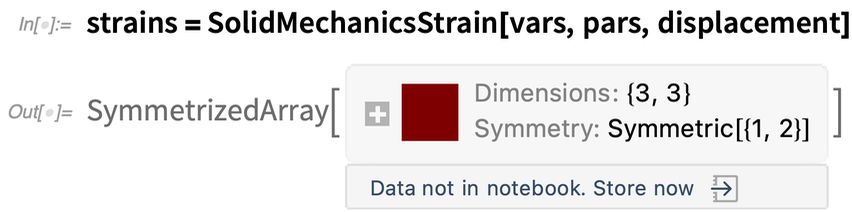✕

And now we can for example find the maximum 3, 3 component of the strain tensor and the position where it’s achieved: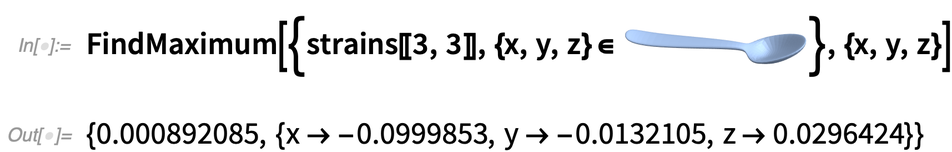✕

How about finding the distribution of values of the strain over the spoon? One easy way to do that is just to sample random points in the spoon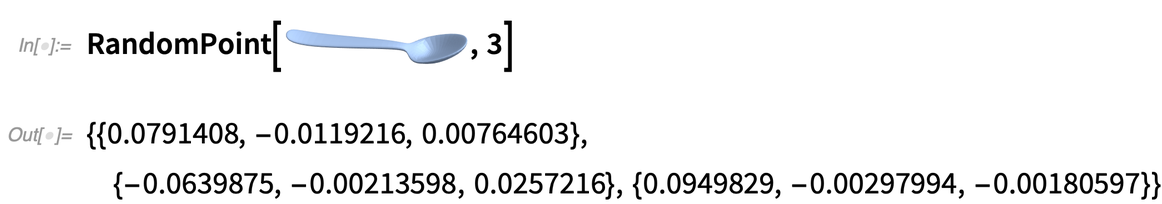✕

and then to make a smoothed histogram of the strains at those points: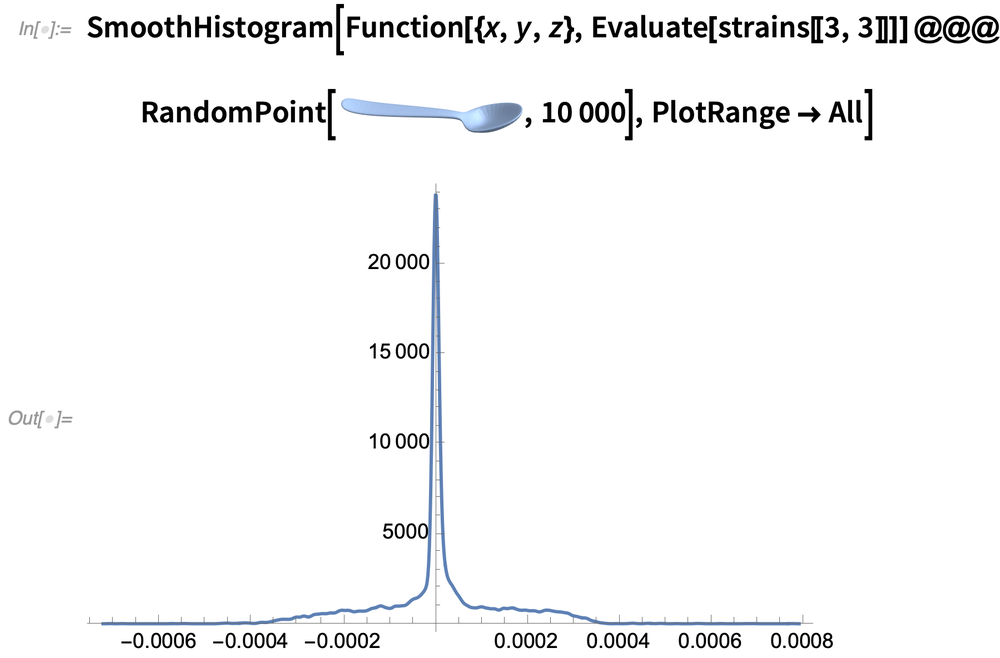✕

(The maximum we saw before is in the tail on the right.)

Solid mechanics is a complicated area, and what we have in Version 13 is good, industrial-grade technology for handling it. And in fact we have a whole monograph titled “Solid Mechanics Model Verification” that describes how we’ve validated our results. We are also providing a general monograph on solid mechanics that describes how to take particular problems and solve them with our technology stack.

## System Modeling & Control Systems

### Microcontroller Support Goes 32-Bit (December 2020)

You’ve developed a control system or signal processing in Wolfram Language. Now how do you deploy it to a piece of standalone electronics? In Version 12.0 we introduced the Microcontroller Kit for compiling from symbolic Wolfram Language structures directly to microcontroller code.

We’ve had lots of feedback on this, asking us to expand the range of microcontrollers that we support. So in Version 12.2 I’m happy to say that we’re adding support for 36 new microcontrollers, particularly 32-bit ones: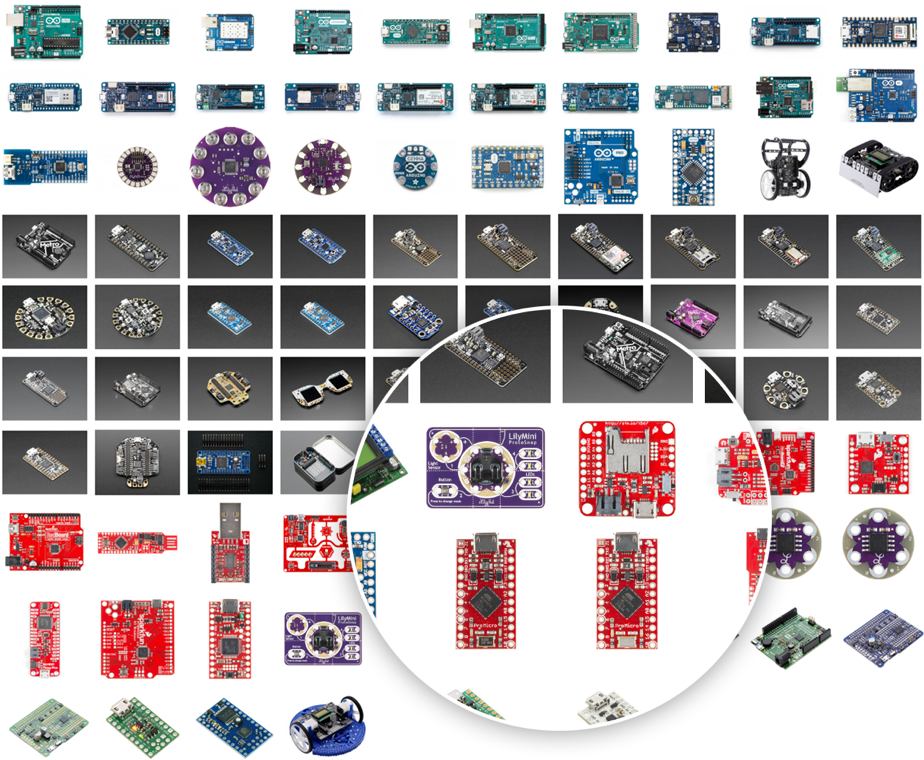Here’s an example in which we deploy a symbolically defined digital filter to a particular kind of microcontroller, showing the simplified C source code generated for that particular microcontroller:✕ `Needs["MicrocontrollerKit`"]`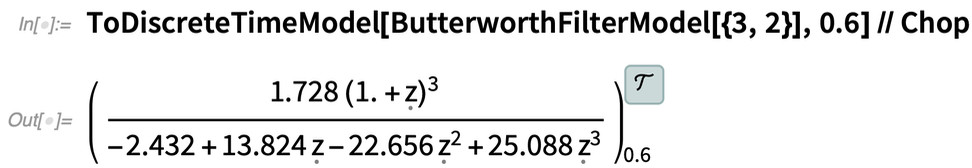✕ `ToDiscreteTimeModel[ButterworthFilterModel[{3, 2}], 0.6] // Chop`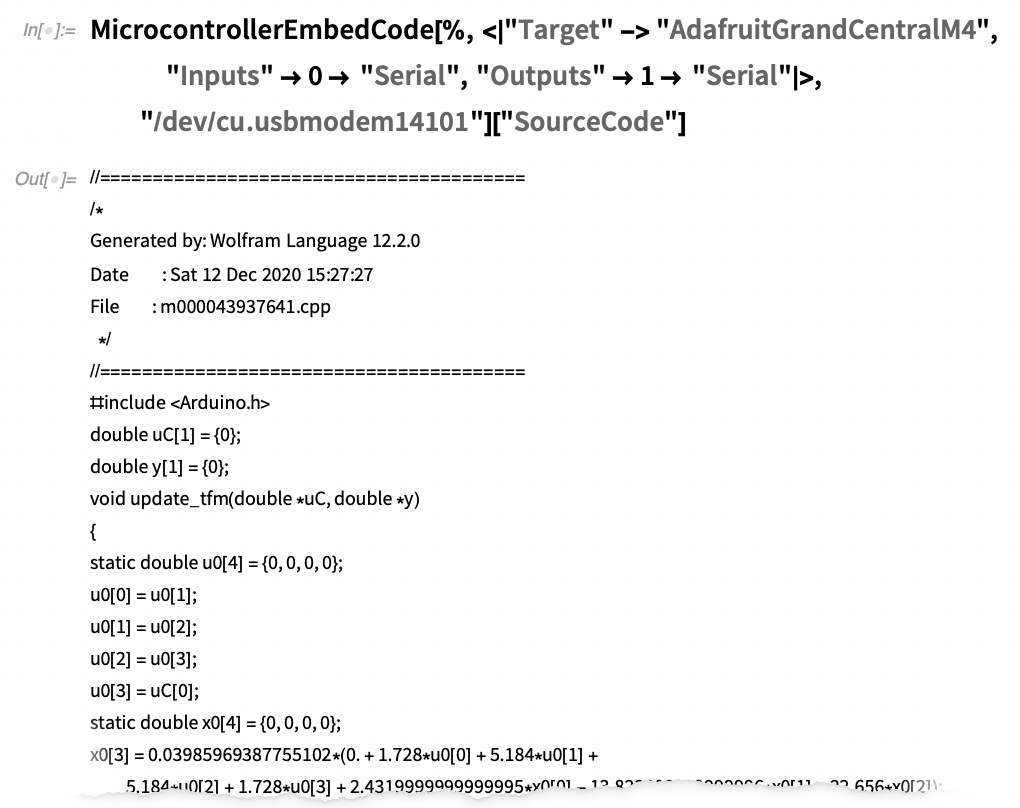✕ ```MicrocontrollerEmbedCode[%, <|"Target" -> "AdafruitGrandCentralM4", "Inputs" -> 0 -> "Serial", "Outputs" -> 1 -> "Serial"|>, "/dev/cu.usbmodem14101"]["SourceCode"]```

### Closing the Loop for Control Systems (May 2021)

It’s something we’ve been working towards for more than a decade. How do we connect our capabilities for representing and simulating large-scale engineering and other systems to our capabilities in control theory? Or, in particular, how can we use our control systems capabilities to create practical designs that can be directly deployed in engineering systems? Version 12.3 takes some important steps in answering this, and developing what’s increasingly a fully automated workflow for control systems design.

Let’s start by importing a model that was created in Wolfram System Modeler. In this particular case, it’s a simple model for a submarine: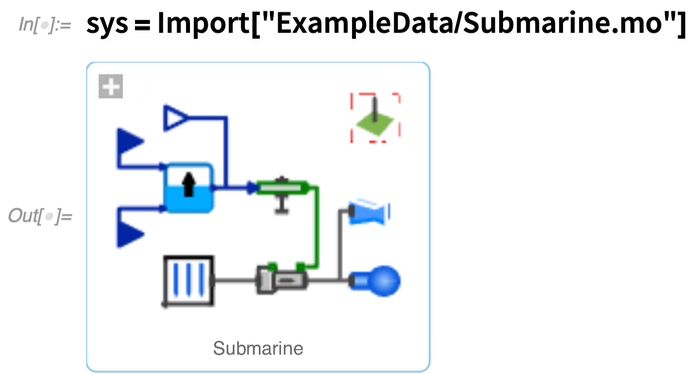✕ `sys = Import["ExampleData/Submarine.mo"]`

Given the model (which in this case consists of more than 300 differential-algebraic equations) we can compute the behavior of the system in different situations. Like here’s a plot of how our model submarine responds to an impulse force—basically showing that the depth of the submarine exhibits damped oscillations: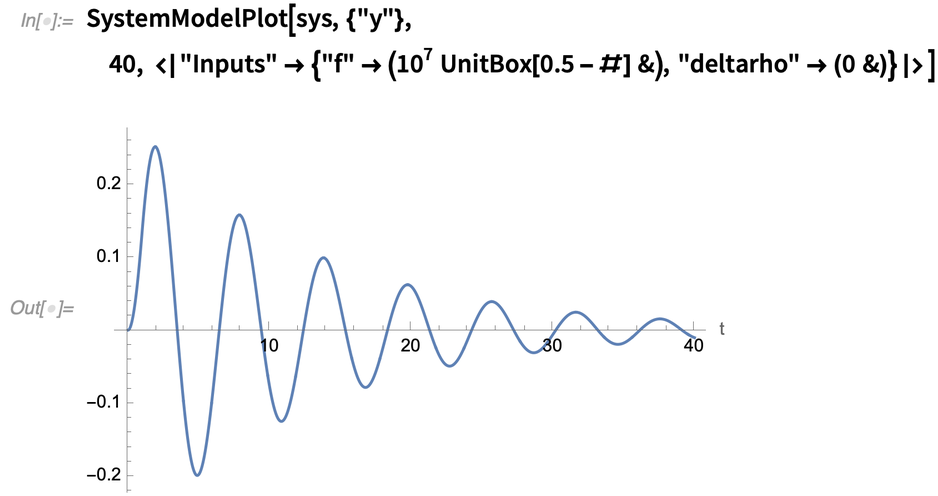✕ ```SystemModelPlot[sys, {"y"}, 40, <| "Inputs" -> {"f" -> (10^7 UnitBox[0.5 - #] &), "deltarho" -> (0 &)}|>]```

But now the question is: how can we control the submarine to prevent those oscillations? Basically we want to set up a closed loop in which a controller will take the observed behavior of the submarine and modify its dynamics to make it stable and well damped, say characterized by particular eigenvalues.

So given the underlying system model, how can we design that controller? Well, in Version 12.3 we’ve managed to get it down to just a couple of functions. First we give the model and parameters that are going to be controlled, and specify our design goal by giving the eigenvalues we want: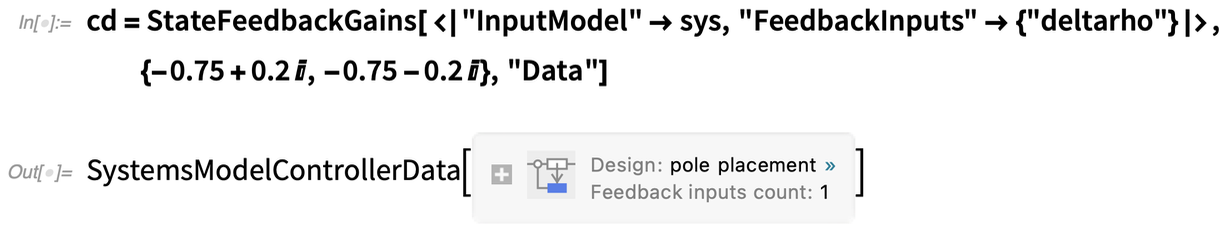✕ ```cd = StateFeedbackGains[<|"InputModel" -> sys, "FeedbackInputs" -> {"deltarho"}|>, {-0.75 + 0.2 I, -0.75 - 0.2 I}, "Data"]```

Now we can take this controller and connect it into our system model: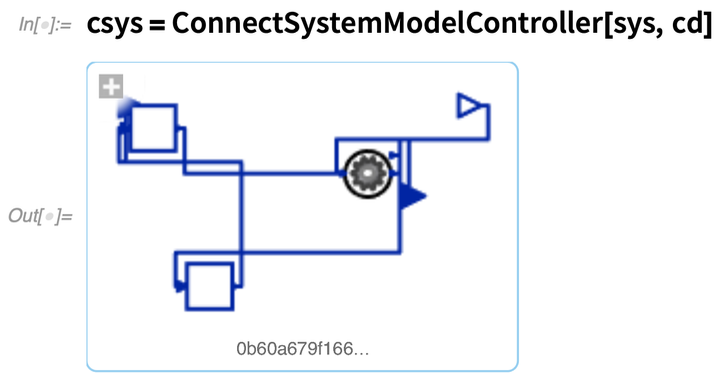✕ `csys = ConnectSystemModelController[sys, cd]`

As the diagram indicates, this is now a closed-loop system (the original system model has been elided into the gray circle). So now we can look at the behavior of this closed-loop system, given for example the same input as before: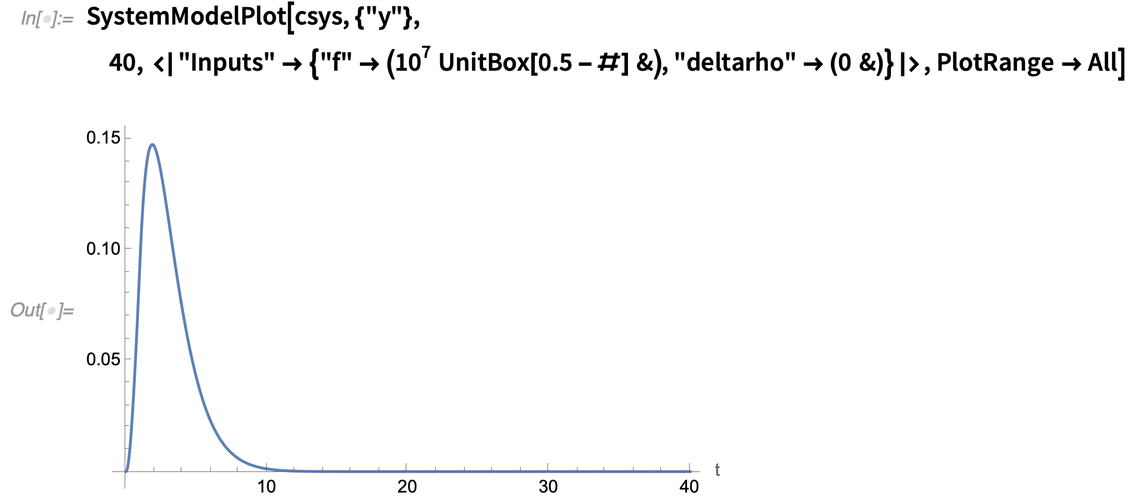✕ ```SystemModelPlot[csys, {"y"}, 40, <| "Inputs" -> {"f" -> (10^7 UnitBox[0.5 - #] &), "deltarho" -> (0 &)}|>, PlotRange -> All]```

Now there are no oscillations; our controller successfully damped them out and “rejected the disturbance”.

So how did this work? Well, as is typical in this type of control systems design, we first found a linearization of the underlying model, appropriate for the domain in which we were going to be operating: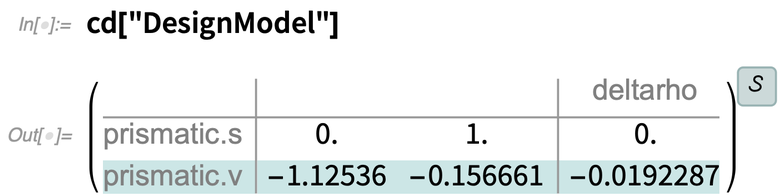✕ `cd["DesignModel"]`

We can get out the eigenvalues of this linearized model: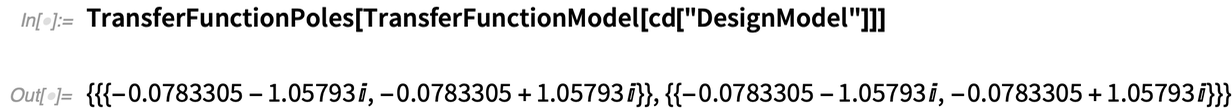✕ `TransferFunctionPoles[TransferFunctionModel[cd["DesignModel"]]]`

The goal of the controller is to shift these to the desired design location: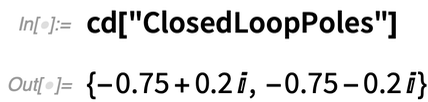✕ `cd["ClosedLoopPoles"]`

So what actually is the controller that was found? Here it is as a nonlinear state space model: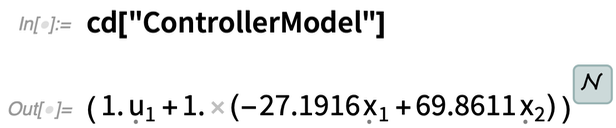✕ `cd["ControllerModel"]`

And now it’s ready to actually deploy. And for example, we can compile the controller for an Arduino: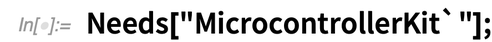✕ `Needs["MicrocontrollerKit`"];`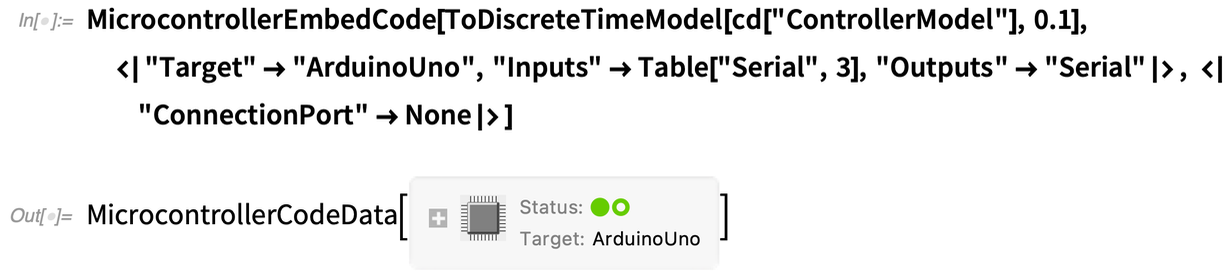✕ ```MicrocontrollerEmbedCode[ ToDiscreteTimeModel[cd["ControllerModel"], 0.1], <|"Target" -> "ArduinoUno", "Inputs" -> Table["Serial", 3], "Outputs" -> "Serial"|>, <|"ConnectionPort" -> None|>]```

And here’s the actual Arduino C source code: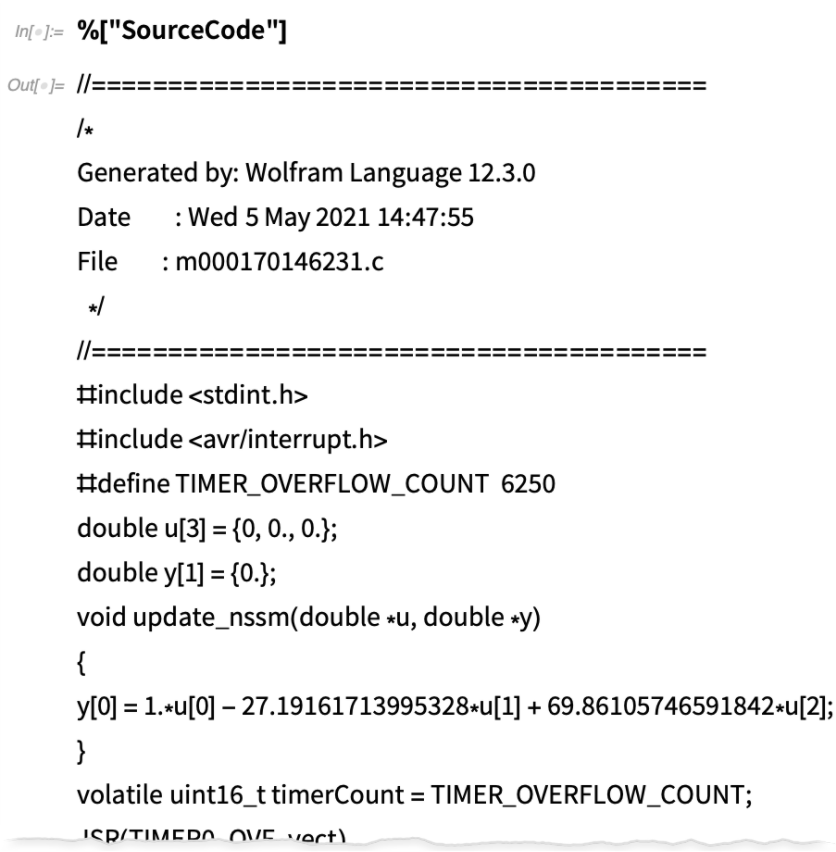✕ `%["SourceCode"]`

Needless to say, for a real submarine, one wouldn’t use an Arduino Uno (though that would probably be just fine for a toy submarine). But the point here is that in Version 12.3 we now have a remarkably automated workflow for going from a sophisticated system model to a control system.

### Automating the Problem of Tracking for Robots and More (December 2021)

Designing control systems is a complicated matter. First, you have to have a model for the system you’re trying to control. Then you have to define the objectives for the controller. And then you have to actually construct a controller that achieves those objectives. With the whole stack of technology in Wolfram Language and Wolfram System Modeler we’re getting to the point where we have an unprecedentedly automated pipeline for doing these things.

Version 13.0 specifically adds capabilities for designing controllers that make a system track a specified signal—for example having a robot that follows a particular trajectory.

Let’s consider a very simple robot that consists of a moving cart with a pointer attached: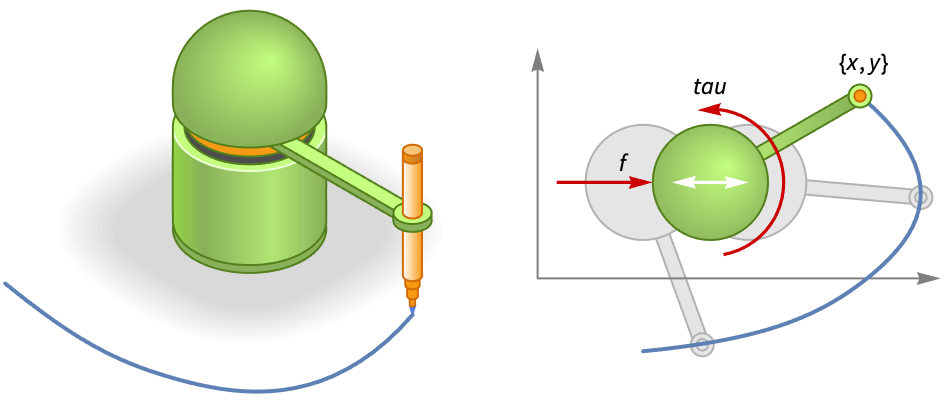First, we need a model for the robot, and this we can create in Wolfram System Modeler (or import as a Modelica model):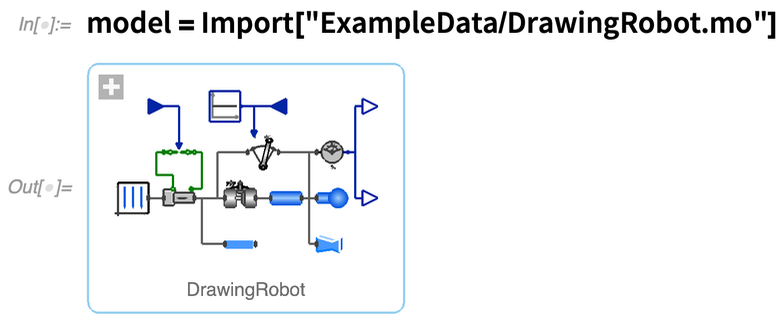✕

Our goal now is to set up a way to “drive” the input variables for the robot (the force moving the cart, and the torque for the pointer)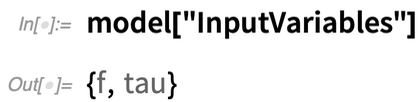✕

in order to achieve certain behavior for the output variables (the position of the end of the pointer):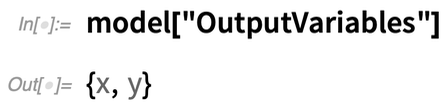✕

Here’s a curve that we want the end of the pointer to follow over time: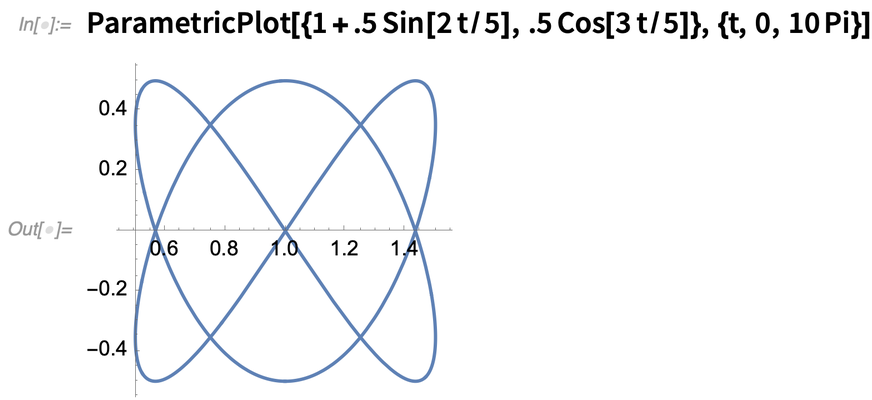✕

Now we want to actually construct the controller—and this is where one needs to know a certain amount about control theory. Here we’re going to use the method of pole placement to create our controller. And we’re going to use the new capability of Version 13.0 to be able to design a “tracking controller” that tracks specified outputs (yes, to set those numbers you have to know about control theory):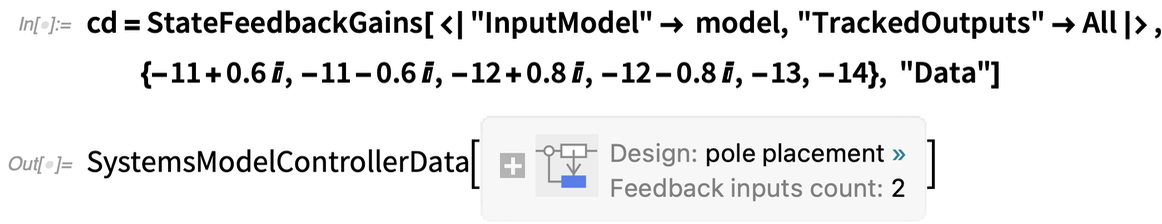✕

Now we have to make the closed-loop system that includes the robot and its controller: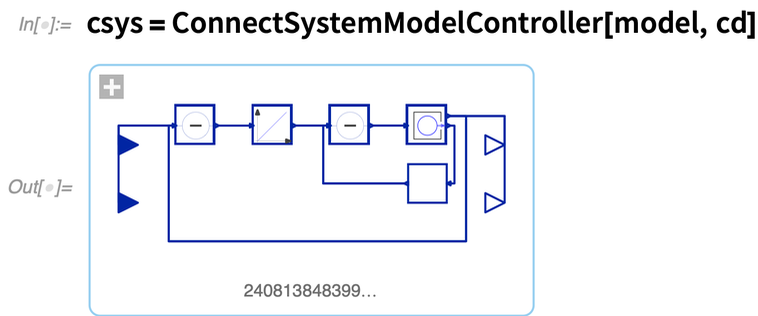✕

And now we can simulate the behavior of this whole system, giving lists of the x and y coordinates of the reference trajectory as input: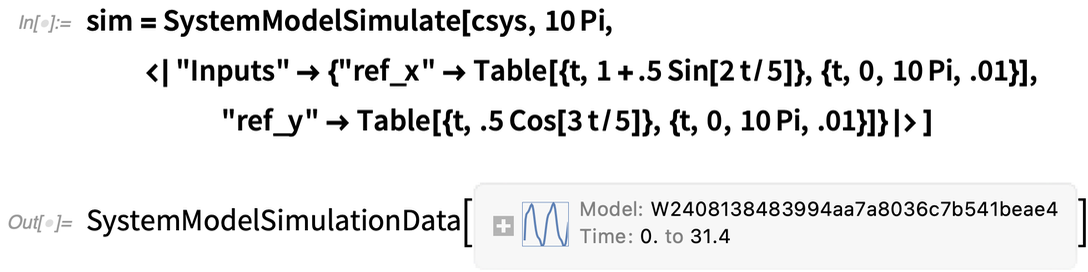✕

And based on this simulation here’s a plot of where the end of the pointer goes: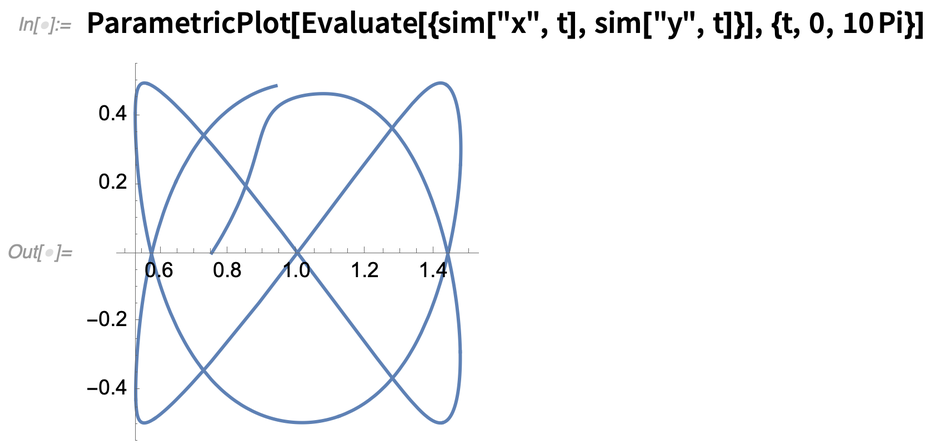✕

After an initial transient, this follows the path we wanted. And, yes, even though this is all a bit complicated, it’s unbelievably simpler than it would be if we were directly using real hardware, rather than doing theoretical “model-based” design.